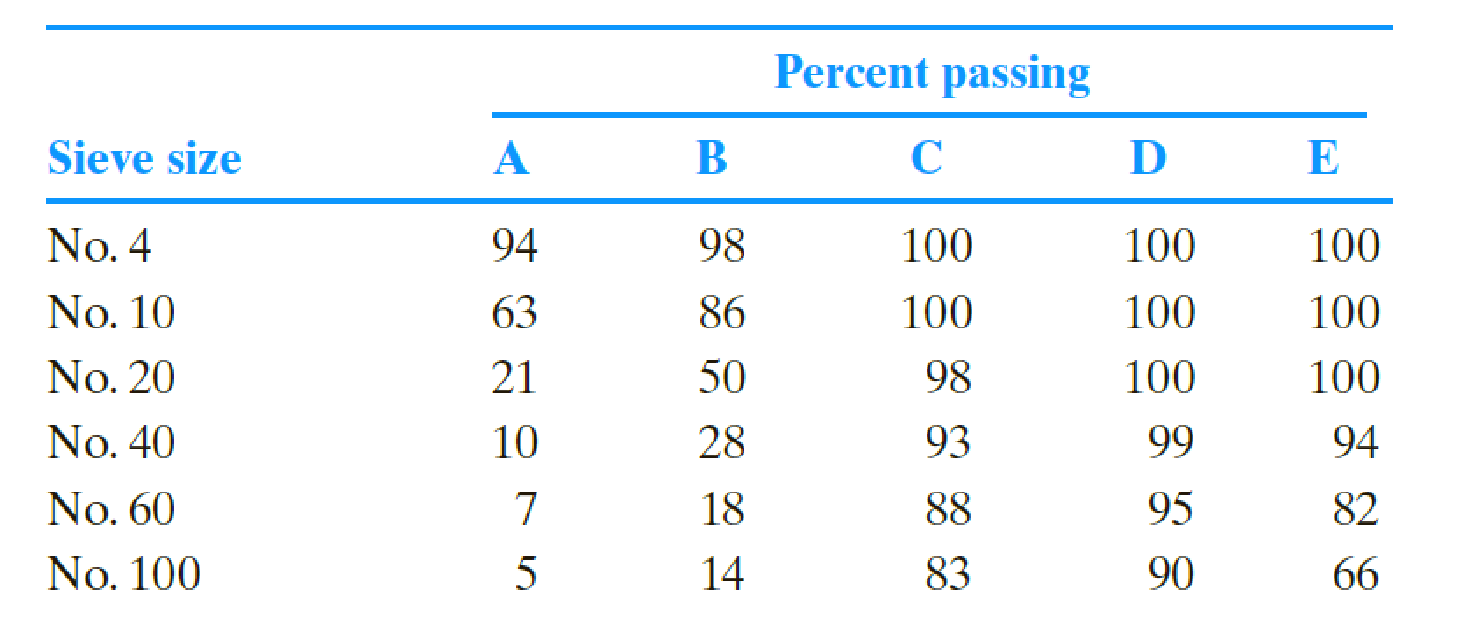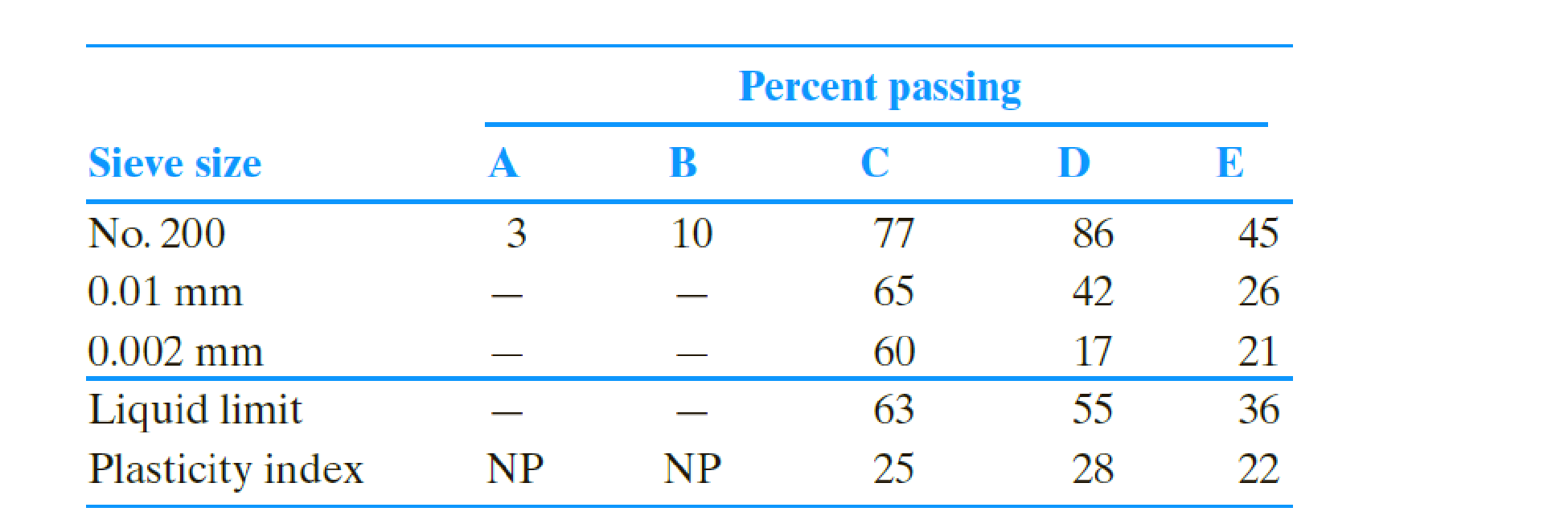Chapter 5, Problem 5.7PPrinciples of Geotechnical Enginee...

9th Edition
Braja M. Das + 1 other
ISBN: 9781305970939

Solutions

Chapter
SectionPrinciples of Geotechnical Enginee...

9th Edition
Braja M. Das + 1 other
ISBN: 9781305970939
Textbook Problem

Classify the following soils using the Unified soil classification system. Give the group symbols and the group names.To determine

Find the classification of the given soil using unified soil classification system and give the group symbols and group names.

Explanation

Calculation:

For soil A:

Determine the fine fraction as shown below.

Coarsefraction=PercentpassingonNo.200seive (1)

Substitute 3 % for percent retained on No.200 sieve in Equation (1).

Finefraction=3%

Determine the coarse fraction as shown below.

Coarsefraction=100PercentpassingonNo.200seive (2)

Substitute 3 % for percent retained on No.200 sieve in Equation (2).

Coarsefraction=1003=97%

Determine the gravel fraction as shown below.

Gravelfraction=100PercentpassingonNo.4seive (3)

Substitute 94 % for percent passing No.4 sieve in Equation (3).

Gravelfraction=10094=6%

Determine the sand fraction using the relation.

Sandfraction=PercentpassingonNo.4seivePercentpassingonNo.200seive (4)

Substitute 94 % for percent retained on No.4 sieve and 3 % for percent retained on No.200 sieve in Equation (4).

Sandfraction=943=91%

The grains passing through No. 4 Sieve is greater than 50 %. Hence, the soil is a sandy soil.

Sketch the grain size distribution curve as shown in Figure 1.

Refer to Figure 1.

The diameter of the particle corresponding to 60% finer (D60) is 1.84mm.

The diameter of the particle corresponding to 30% finer (D30) is 0.96mm.

The diameter of the particle corresponding to 10% finer (D10) is 0.41mm.

Calculate the uniformity coefficient (Cu) using the relation.

Cu=D60D10 (5)

Substitute 1.84mm for D60 and 0.41mm for D10 in Equation (5).

Cu=1.840.41=4.49

Calculate the coefficient of gradation (Cc) using the relation.

Cc=D302D60×D10 (6)

Substitute 1.84mm for D60, 0.96mm for D30, and 0.41mm for D10 in Equation (6).

Cc=0.9621.84×0.91=1.22

Refer Table 5.2 “Unified soil classification system” in the text book.

The group symbol of the soil can be taken as SP_.

Provide the group name of soil as shown below.

The gravel fraction 6 % is <15%.

Refer Figure 5.4 “Flowchart group names for gravelly and sandy soil” in the text book.

Take the group name as poorly graded sand for the group of SP and gravel fraction of <15%.

Thus, the classification of soil A using unified soil classification system is Poorly graded sand_.

For soil B:

Determine the fine fraction as shown below.

Substitute 10 % for percent retained on No.200 sieve in Equation (1).

Finefraction=10%

Determine the coarse fraction as shown below.

Substitute 10 % for percent retained on No.200 sieve in Equation (2).

Coarsefraction=10010=90%

Determine the gravel fraction as shown below.

Substitute 98 % for percent passing No.4 sieve in Equation (3).

Gravelfraction=10098=2%

Determine the sand fraction using the relation.

Substitute 98 % for percent retained on No.4 sieve and 10 % for percent retained on No.200 sieve in Equation (4).

Sandfraction=9810=88%

The grains passing through No. 4 Sieve is greater than 50 %. Hence, the soil is a sandy soil.

The fine fraction 10 % is lies between 5 % to 12 %. Hence, the symbol of soil is a dual symbol.

Refer to Figure 1.

The diameter of the particle corresponding to 60% finer (D60) is 1.08mm.

The diameter of the particle corresponding to 30% finer (D30) is 0.44mm.

The diameter of the particle corresponding to 10% finer (D10) is 0

Still sussing out bartleby?

Check out a sample textbook solution.

See a sample solution

The Solution to Your Study Problems

Bartleby provides explanations to thousands of textbook problems written by our experts, many with advanced degrees!

Get Started

List and define the common attributes of tasks within a WBS.

Principles of Information Security (MindTap Course List)

What does the term 3 Vs refer to?

Database Systems: Design, Implementation, & Management

Explain Appleâ€™s view of user interface design, especially for apps.

Systems Analysis and Design (Shelly Cashman Series) (MindTap Course List)

Which Linux utility provides output similar to Wiresharks?

Network+ Guide to Networks (MindTap Course List)

Describe the motion of the electrode in keyhole welding.

Welding: Principles and Applications (MindTap Course List)

How do the responsibilities of a project manager differ from those of a project leader? (517)

Enhanced Discovering Computers 2017 (Shelly Cashman Series) (MindTap Course List)